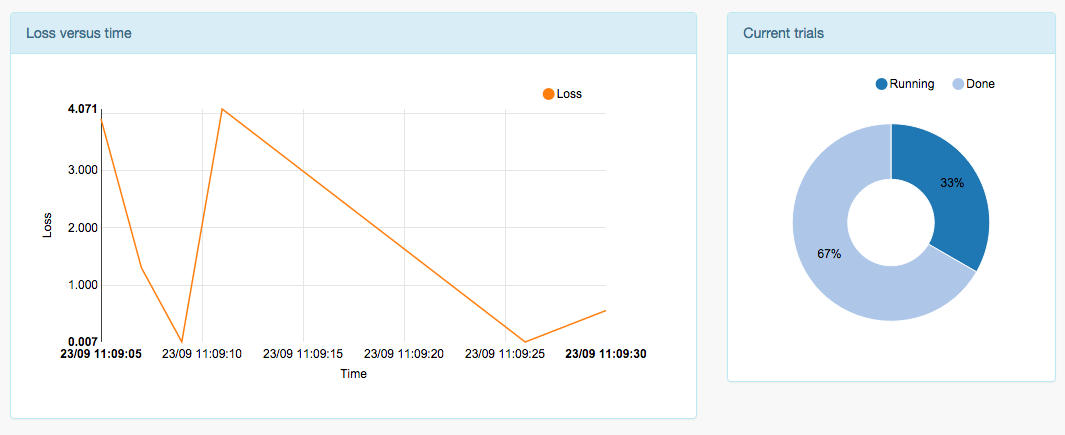## Get started

Finding the best hyperparameters for your machine learning algorithms is a difficult job.
Oscar has been built to relieve your datascientist from this tedious task.

He can be up and runnig for you in 5 minutes:

### 1. Install Oscar's client

```pip install git+git://github.com/sensout/Oscar-Python.git
```
```luarocks install Oscar --from=https://raw.githubusercontent.com/sensout/Oscar-Lua/master/
```

### 3. Run your first experiment

```# Get Oscar
from Oscar import Oscar
scientist = Oscar('API_ACCESS_TOKEN')

experiment = {'name':'Square', 'parameters':{'x' : {'min': -10, 'max' : 10}}}

for i in range(1, 10):
# Get next parameters to try from Oscar
job = scientist.suggest(experiment)
print job

# Run you complex, time-consuming algorithm
import math
loss = math.pow(job['x'], 2)

# Tell Oscar the result
scientist.update(job, {'loss' : loss})
```
```-- Get Oscar
local Oscar = require('Oscar')
local scientist = Oscar('API_ACCESS_TOKEN')

local experiment = {name='Square', parameters={x = {min = -10, max = 10}}}

for i = 1, 10 do
-- Get next parameters to try from Oscar
local job = scientist:suggest(experiment)
print(job)

-- Run you complex, time-consuming algorithm
local loss = math.pow(job.x, 2)

-- Tell Oscar the result
scientist:update(job, {loss = loss})
end
```

After a few runs you should see some interesting insights about your experiment in the Trials panel.## Experiment

### The `experiment` object

The `experiment` object describes your experiment to Oscar when you call the `suggest(experiment)` method.

It has a JSON-like format (dict in Python/table in Lua) with mandatory and optional key-value pairs:

Example with options
```experiment = {
'name' : 'Square',
'description' : 'This is a very simple experiment',
'parameters' : ...,
'resume' : True
}
job = scientist.suggest(experiment)
```
```experiment = {
name = 'Square',
description = 'This is a very simple experiment',
parameters = ...,
resume : true
}
job = scientist:suggest(experiment)
```
KeyDescriptionRequiredPossible valuesDefault value
`name` This is the name of your experiment Yes `string` None
`description` A description of your experiment for easier monitoring in the dashboard No `string` `''`
`parameters` Describes the pararameters of your experiments Yes see bellow None
`resume` Resume an experiment with the same name if True
Overrides it if False
No `boolean` `true`

### The `parameters` object

The `parameters` object has the same JSON-like format as the `experiment` object.
It describes the hyper parameters space of your machine learning algorithm.

Besides describing the range of each hyper parameter you can describe its probabilistic distribution.
You can see this as a way to convey your experience and intuition to Oscar to speed up the hyper parameters space exploration.

Each hyper parameter is defined as a key-value pair in the `parameters` object:

• The key is the name of the hyper parameter

• The value is another key-value pair object describing the space of the hyper parameter.

#### Categorical parameter

If your parameter takes discrete values which are not ordered or related, it is a categorical parameter.

Describe it with an array of its possible values.

Example: Try different activation functions in a neural network
```'parameters' : {'activation' : ['relu', 'tanh']}
```
```parameters = {activation = {'relu', 'tanh'}}
```

#### Uniformly distributed parameter

If your parameter takes continous or discrete values which are uniformly distributed, you must specify its range with the `min` and `max` keys.

If your parameter takes dicrete values, you can specify the step size with the `step` key.

If your parameter has a log uniform distribution, use the `log` key.

Example: Try different number of layers and various batch size in a neural network
```'parameters' : {
'layers' : {'min' : 1, 'max' : 3, 'step' : 1},
'batch_size' : {'min' : 10, 'max' : 100, 'step' : 10}
}
```
```parameters = {
layers = {min = 1, max = 3, step = 1},
batch_size : {min = 10, max = 100, step = 10}
}
```

#### Normally distributed parameter

If your parameter takes continous or discrete values which are normaly distributed, you must specify its mean and standard deviation with the `mu` and `sigma` keys.

If your parameter takes dicrete values, you can specify the step size with the `step` key.

If your parameter has a log normal distribution, use the `log` key.

### Pushing the experiment result

Once you've run your experiment, you must tell Oscar the result so he can take it into account for his next hyper parameter suggestion.

The result of your experiment is encoded in a JSON-like format with the mandatory `loss` key which Oscar will try to minimize (Invert it if you want to maximize an objective).

You can also add any other custom parameters in order to visualize them in the report.

Example: Update for a neural network
```result = {
# We want to optimize the validation loss
'loss' : 2.0,
'val_time' : 5,
'train_loss' : 3.0,
'train_time' : 20
}
scientist.update(job, result)
```
```local result = {
-- We want to optimize the validation loss
loss = 2.0,
val_time = 5,
train_loss = 3.0,
train_time = 20
}
scientist:update(job, result)
```

## Running on a cluster

Oscar is ready to scale and handle many experiments simultaneously.

Define your experiment and install Oscar's client on as many compute nodes as you like.
Then simply run your experiment as long as you want on every node:

```#!/bin/bash

# loop infinitely
while true
do
python experiment.py
done
```
```#!/bin/bash

# loop infinitely
while true
do
th experiment.lua
done
```

### Running in the cloud

Run your experiments in the cloud with a few lines:

Adapt and paste the following script into the User data field of your instance:

```#!/bin/bash

# install the requirements for your experiment
# ex: install theano
# wget http://www.yoursite.com/yourexperiemnt.tar.gz
# tar xvf yourexperiemnt.tar.gz

# install Oscar's Python client
pip install git+git://github.com/sensout/Oscar-Python.git

# loop infinitely
while true
do
python experiment.py
done
```

Example if requested.

## Handling experiment interruption

Oscar can cope with experiment interruption and resume it after a node reboot for example.

This gives you the ability to reduce your cost by running your long duration experiments on a low cost infrastructure such as AWS EC2 Spot instances.